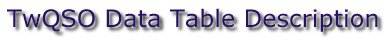A Description of the TwQSO = Q0957+561 A,B Data File

An accompanying file lists the optical data for the 2-image gravitationally lensed system Q0957+561 A,B, sometimes called simply the TwQSO. This text file simply describes the columns of the table, and gives some background information about it.

Successive columns list the calendar date of the observation as year, month, and day. The next entry is the Julian date, with the initial digits 24 deleted. Thus the full Julian date of the first observation is 2,444,193.9. The next column lists the A image brightness in R filter magnitudes and the next column lists the B image brightness, corrected for the brightness of the lens galaxy, taken to be R=18.34 in the B aperture. The next column lists the source of the photometry (S = Mt Hopkins data, V= Vanderriest et al, 1989, H = Hamburg), the next column gives the A magnitude error in millimagnitudes, and the final column gives the B image error in millimagnitudes.

To hopefully clarify, I will give as an example a reading of the first line. An observation was made on 16 Nov 1979, which is Julian date 2444193.9. The measured A image brightness behind an R filter with a standard R magnitude zero point was R = 16.62. The B (southern) image had an R magnitude of 16.62, after correction was made for the 18.34 magnitude lens galaxy light that was also in the analyzing aperture. The observation was made by me at the F. L. Whipple Observatory on Mt Hopkins. The error of the A observation was 10 millimagnitudes, so the observed brightness and one sigma error bar were R = 16.62 +/- .01 mag. Similarly the corrected B magnitude and one sigma error bar were R = 16.96 +/- .01 mag.

Any questions?

[ Main ]